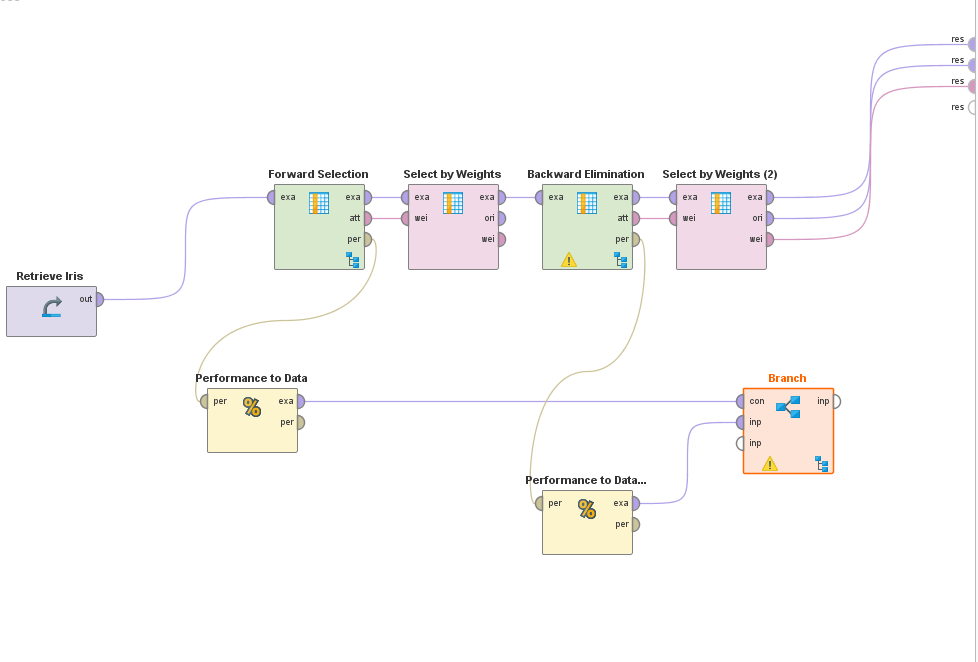# compare 2 performance values and branch process if one better than the other

Member Posts: 344Unicorn
edited July 2019 in Help

hi,

I am trying a combined Forward Selection / Backward Elimination approach for Feature Selection, currently, I want to branch and continue into forward Selection, if the performance from the previous backward selection gave no better performance results than the forward selection. otherwise, if it got better with backward selection, I want to continue backward selection until performance gets worse than the best currently backward selection performance.

How can I branch the process, based on some performance condition?

here is my process:I know I would of course put the whole process into the branch subprocess, but this is only for testing / concept purposes to get better understanding what I meanand furthermore, I would like to know if I can do this in kind of a loop style, e.g feed into forward selecion the results from the previous backward selection, or if I would have to

use multiple forward / backward selections in a row, e.g 5 of them If I want to do it 5 times...

or is it possible in a loop like I said, and then define some aborting condition, e.g after 5 loops of if performance does not increase anymore...

Tagged:

• Administrator, Moderator, Employee, RapidMiner Certified Analyst, RapidMiner Certified Expert, University Professor Posts: 2,224RM Data Scientist

Moin Fred,

attached is the process like i would built it. Truth said, it feels a bit complicated for the task. I will check if there is a better way to do this.

~Martin

`<?xml version="1.0" encoding="UTF-8"?><process version="7.3.000">  <context>    <input/>    <output/>    <macros/>  </context>  <operator activated="true" class="process" compatibility="7.3.000" expanded="true" name="Process">    <process expanded="true">      <operator activated="true" class="generate_data" compatibility="7.3.000" expanded="true" height="68" name="Generate Data" width="90" x="45" y="187">        <parameter key="target_function" value="complicated function2"/>        <description align="center" color="transparent" colored="false" width="126">Generate Random Data</description>      </operator>      <operator activated="true" class="concurrency:cross_validation" compatibility="7.3.000" expanded="true" height="145" name="Cross Validation" width="90" x="246" y="34">        <process expanded="true">          <operator activated="true" class="h2o:gradient_boosted_trees" compatibility="7.3.000" expanded="true" height="103" name="Gradient Boosted Trees" width="90" x="112" y="34">            <list key="expert_parameters"/>          </operator>          <connect from_port="training set" to_op="Gradient Boosted Trees" to_port="training set"/>          <connect from_op="Gradient Boosted Trees" from_port="model" to_port="model"/>          <portSpacing port="source_training set" spacing="0"/>          <portSpacing port="sink_model" spacing="0"/>          <portSpacing port="sink_through 1" spacing="0"/>        </process>        <process expanded="true">          <operator activated="true" class="apply_model" compatibility="7.3.000" expanded="true" height="82" name="Apply Model" width="90" x="45" y="34">            <list key="application_parameters"/>          </operator>          <operator activated="true" class="performance_regression" compatibility="7.3.000" expanded="true" height="82" name="Performance" width="90" x="246" y="34"/>          <connect from_port="model" to_op="Apply Model" to_port="model"/>          <connect from_port="test set" to_op="Apply Model" to_port="unlabelled data"/>          <connect from_op="Apply Model" from_port="labelled data" to_op="Performance" to_port="labelled data"/>          <connect from_op="Performance" from_port="performance" to_port="performance 1"/>          <portSpacing port="source_model" spacing="0"/>          <portSpacing port="source_test set" spacing="0"/>          <portSpacing port="source_through 1" spacing="0"/>          <portSpacing port="sink_test set results" spacing="0"/>          <portSpacing port="sink_performance 1" spacing="0"/>          <portSpacing port="sink_performance 2" spacing="0"/>        </process>        <description align="center" color="transparent" colored="false" width="126">Run Parallel X-Val</description>      </operator>      <operator activated="true" class="performance_to_data" compatibility="7.3.000" expanded="true" height="82" name="Performance to Data" width="90" x="380" y="238"/>      <operator activated="true" class="concurrency:cross_validation" compatibility="7.3.000" expanded="true" height="145" name="Cross Validation (2)" width="90" x="447" y="34">        <process expanded="true">          <operator activated="false" class="h2o:gradient_boosted_trees" compatibility="7.3.000" expanded="true" height="103" name="Gradient Boosted Trees (2)" width="90" x="112" y="289">            <list key="expert_parameters"/>          </operator>          <operator activated="true" class="h2o:generalized_linear_model" compatibility="7.3.000" expanded="true" height="103" name="Generalized Linear Model" width="90" x="179" y="34">            <list key="beta_constraints"/>            <list key="expert_parameters"/>          </operator>          <connect from_port="training set" to_op="Generalized Linear Model" to_port="training set"/>          <connect from_op="Generalized Linear Model" from_port="model" to_port="model"/>          <portSpacing port="source_training set" spacing="0"/>          <portSpacing port="sink_model" spacing="0"/>          <portSpacing port="sink_through 1" spacing="0"/>        </process>        <process expanded="true">          <operator activated="true" class="apply_model" compatibility="7.3.000" expanded="true" height="82" name="Apply Model (2)" width="90" x="45" y="34">            <list key="application_parameters"/>          </operator>          <operator activated="true" class="performance_regression" compatibility="7.3.000" expanded="true" height="82" name="Performance (2)" width="90" x="246" y="34"/>          <connect from_port="model" to_op="Apply Model (2)" to_port="model"/>          <connect from_port="test set" to_op="Apply Model (2)" to_port="unlabelled data"/>          <connect from_op="Apply Model (2)" from_port="labelled data" to_op="Performance (2)" to_port="labelled data"/>          <connect from_op="Performance (2)" from_port="performance" to_port="performance 1"/>          <portSpacing port="source_model" spacing="0"/>          <portSpacing port="source_test set" spacing="0"/>          <portSpacing port="source_through 1" spacing="0"/>          <portSpacing port="sink_test set results" spacing="0"/>          <portSpacing port="sink_performance 1" spacing="0"/>          <portSpacing port="sink_performance 2" spacing="0"/>        </process>        <description align="center" color="transparent" colored="false" width="126">Run Parallel X-Val</description>      </operator>      <operator activated="true" class="performance_to_data" compatibility="7.3.000" expanded="true" height="82" name="Performance to Data (2)" width="90" x="581" y="85"/>      <operator activated="true" class="join" compatibility="7.3.000" expanded="true" height="82" name="Join" width="90" x="715" y="136">        <parameter key="remove_double_attributes" value="false"/>        <parameter key="use_id_attribute_as_key" value="false"/>        <list key="key_attributes">          <parameter key="Criterion" value="Criterion"/>        </list>      </operator>      <operator activated="true" class="generate_attributes" compatibility="7.3.000" expanded="true" height="82" name="Generate Attributes" width="90" x="849" y="136">        <list key="function_descriptions">          <parameter key="delta_perf" value="Value-Value_from_ES2"/>        </list>      </operator>      <operator activated="true" class="branch" compatibility="7.3.000" expanded="true" height="124" name="Branch" width="90" x="983" y="85">        <parameter key="condition_type" value="attribute_value_filter"/>        <parameter key="condition_value" value="delta_perf &gt;0"/>        <parameter key="expression" value="delta_perf&gt;0"/>        <process expanded="true">          <connect from_port="input 1" to_port="input 1"/>          <portSpacing port="source_condition" spacing="0"/>          <portSpacing port="source_input 1" spacing="0"/>          <portSpacing port="source_input 2" spacing="0"/>          <portSpacing port="source_input 3" spacing="0"/>          <portSpacing port="sink_input 1" spacing="0"/>          <portSpacing port="sink_input 2" spacing="0"/>        </process>        <process expanded="true">          <connect from_port="input 2" to_port="input 1"/>          <portSpacing port="source_condition" spacing="0"/>          <portSpacing port="source_input 1" spacing="0"/>          <portSpacing port="source_input 2" spacing="0"/>          <portSpacing port="source_input 3" spacing="0"/>          <portSpacing port="sink_input 1" spacing="0"/>          <portSpacing port="sink_input 2" spacing="0"/>        </process>      </operator>      <connect from_op="Generate Data" from_port="output" to_op="Cross Validation" to_port="example set"/>      <connect from_op="Cross Validation" from_port="model" to_op="Branch" to_port="input 2"/>      <connect from_op="Cross Validation" from_port="example set" to_op="Cross Validation (2)" to_port="example set"/>      <connect from_op="Cross Validation" from_port="performance 1" to_op="Performance to Data" to_port="performance vector"/>      <connect from_op="Performance to Data" from_port="example set" to_op="Join" to_port="right"/>      <connect from_op="Cross Validation (2)" from_port="model" to_op="Branch" to_port="input 1"/>      <connect from_op="Cross Validation (2)" from_port="performance 1" to_op="Performance to Data (2)" to_port="performance vector"/>      <connect from_op="Performance to Data (2)" from_port="example set" to_op="Join" to_port="left"/>      <connect from_op="Join" from_port="join" to_op="Generate Attributes" to_port="example set input"/>      <connect from_op="Generate Attributes" from_port="example set output" to_op="Branch" to_port="condition"/>      <connect from_op="Branch" from_port="input 1" to_port="result 1"/>      <portSpacing port="source_input 1" spacing="0"/>      <portSpacing port="sink_result 1" spacing="0"/>      <portSpacing port="sink_result 2" spacing="0"/>    </process>  </operator></process>`

- Head of Data Science Services at RapidMiner -
Dortmund, Germany